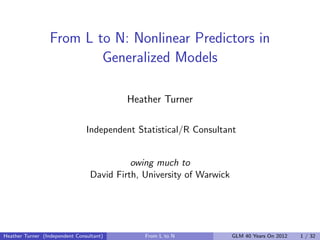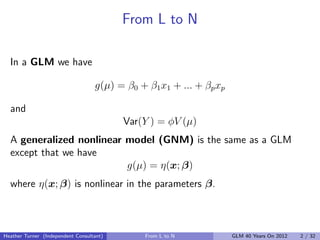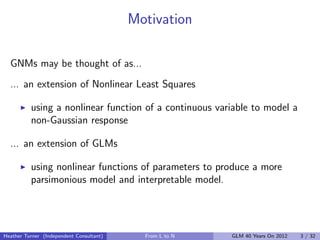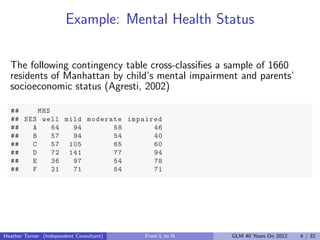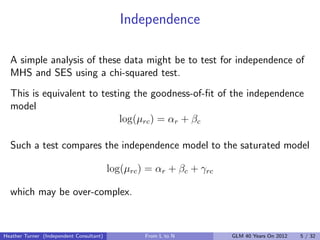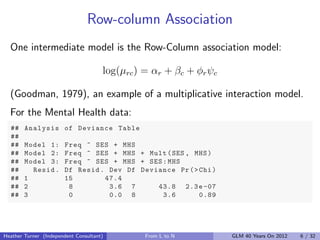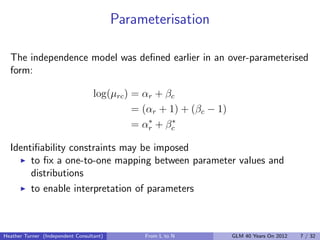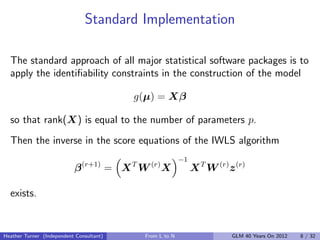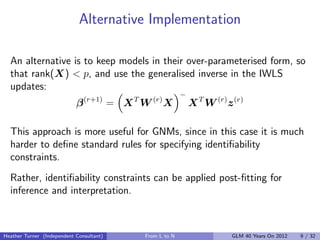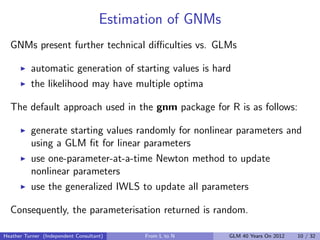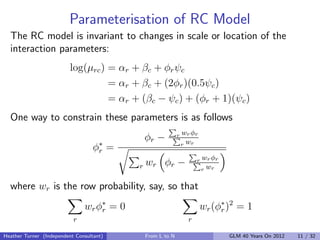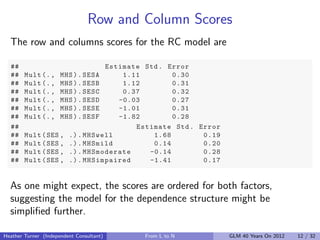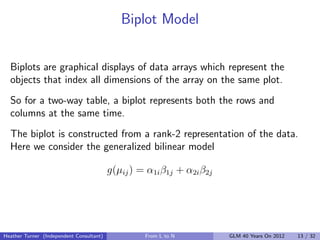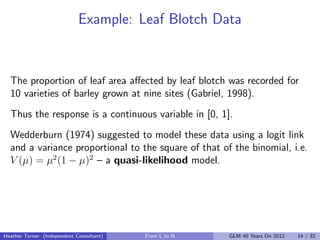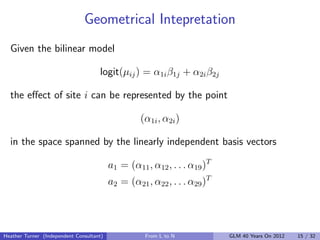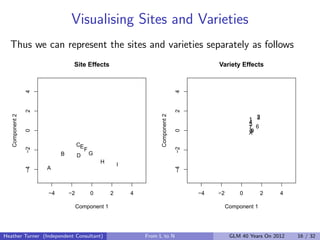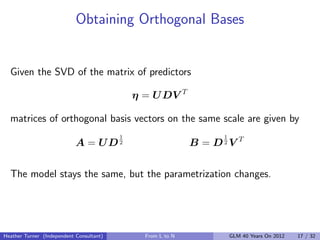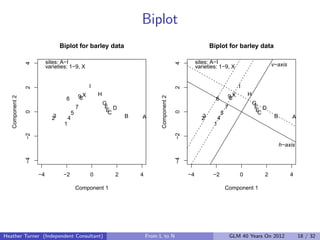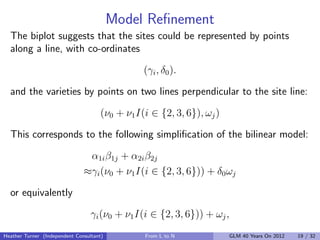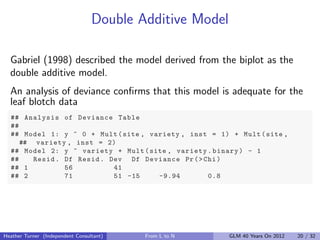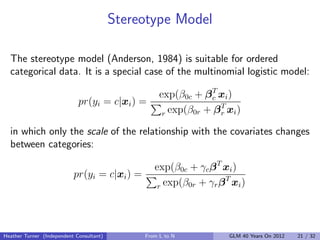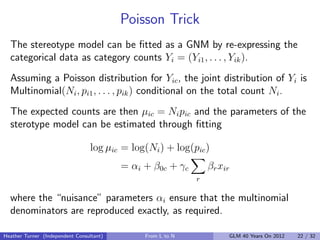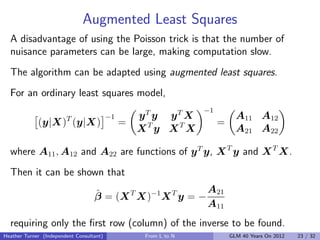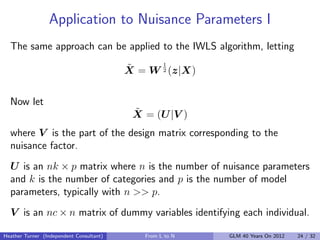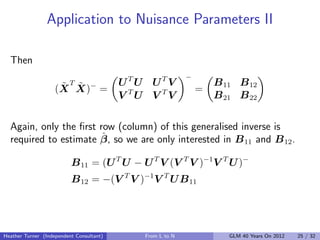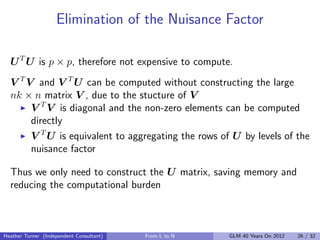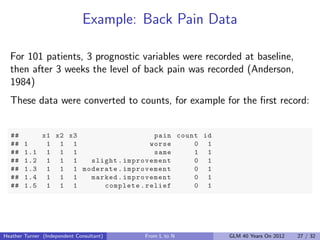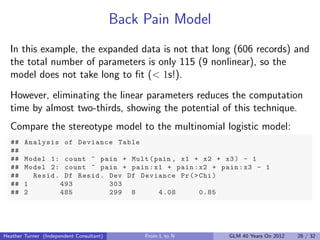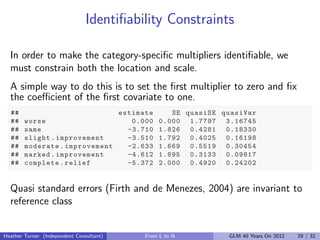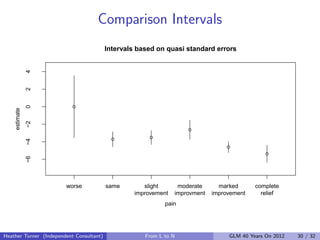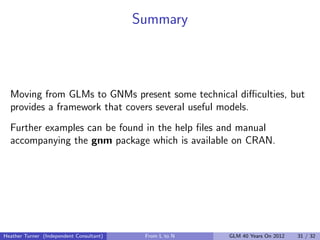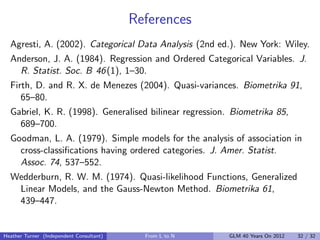1 of 32

### From L to N: Nonlinear Predictors in Generalized Models

1. From L to N: Nonlinear Predictors in Generalized Models Heather Turner Independent Statistical/R Consultant owing much to David Firth, University of Warwick Heather Turner (Independent Consultant) From L to N GLM 40 Years On 2012 1 / 32
2. From L to N In a GLM we have g(µ) = β0 + β1 x1 + ... + βp xp and Var(Y ) = φV (µ) A generalized nonlinear model (GNM) is the same as a GLM except that we have g(µ) = η(x; β) where η(x; β) is nonlinear in the parameters β. Heather Turner (Independent Consultant) From L to N GLM 40 Years On 2012 2 / 32
3. Motivation GNMs may be thought of as... ... an extension of Nonlinear Least Squares using a nonlinear function of a continuous variable to model a non-Gaussian response ... an extension of GLMs using nonlinear functions of parameters to produce a more parsimonious model and interpretable model. Heather Turner (Independent Consultant) From L to N GLM 40 Years On 2012 3 / 32
4. Example: Mental Health Status The following contingency table cross-classiﬁes a sample of 1660 residents of Manhattan by child’s mental impairment and parents’ socioeconomic status (Agresti, 2002) ## MHS ## SES well mild moderate impaired ## A 64 94 58 46 ## B 57 94 54 40 ## C 57 105 65 60 ## D 72 141 77 94 ## E 36 97 54 78 ## F 21 71 54 71 Heather Turner (Independent Consultant) From L to N GLM 40 Years On 2012 4 / 32
5. Independence A simple analysis of these data might be to test for independence of MHS and SES using a chi-squared test. This is equivalent to testing the goodness-of-ﬁt of the independence model log(µrc ) = αr + βc Such a test compares the independence model to the saturated model log(µrc ) = αr + βc + γrc which may be over-complex. Heather Turner (Independent Consultant) From L to N GLM 40 Years On 2012 5 / 32
6. Row-column Association One intermediate model is the Row-Column association model: log(µrc ) = αr + βc + φr ψc (Goodman, 1979), an example of a multiplicative interaction model. For the Mental Health data: ## Analysis of Deviance Table ## ## Model 1: Freq ~ SES + MHS ## Model 2: Freq ~ SES + MHS + Mult ( SES , MHS ) ## Model 3: Freq ~ SES + MHS + SES : MHS ## Resid . Df Resid . Dev Df Deviance Pr ( > Chi ) ## 1 15 47.4 ## 2 8 3.6 7 43.8 2.3 e -07 ## 3 0 0.0 8 3.6 0.89 Heather Turner (Independent Consultant) From L to N GLM 40 Years On 2012 6 / 32
7. Parameterisation The independence model was deﬁned earlier in an over-parameterised form: log(µrc ) = αr + βc = (αr + 1) + (βc − 1) ∗ ∗ = αr + βc Identiﬁability constraints may be imposed to ﬁx a one-to-one mapping between parameter values and distributions to enable interpretation of parameters Heather Turner (Independent Consultant) From L to N GLM 40 Years On 2012 7 / 32
8. Standard Implementation The standard approach of all major statistical software packages is to apply the identiﬁability constraints in the construction of the model g(µ) = Xβ so that rank(X) is equal to the number of parameters p. Then the inverse in the score equations of the IWLS algorithm −1 β (r+1) = X T W (r) X X T W (r) z (r) exists. Heather Turner (Independent Consultant) From L to N GLM 40 Years On 2012 8 / 32
9. Alternative Implementation An alternative is to keep models in their over-parameterised form, so that rank(X) < p, and use the generalised inverse in the IWLS updates: − β (r+1) = X T W (r) X X T W (r) z (r) This approach is more useful for GNMs, since in this case it is much harder to deﬁne standard rules for specifying identiﬁability constraints. Rather, identiﬁability constraints can be applied post-ﬁtting for inference and interpretation. Heather Turner (Independent Consultant) From L to N GLM 40 Years On 2012 9 / 32
10. Estimation of GNMs GNMs present further technical diﬃculties vs. GLMs automatic generation of starting values is hard the likelihood may have multiple optima The default approach used in the gnm package for R is as follows: generate starting values randomly for nonlinear parameters and using a GLM ﬁt for linear parameters use one-parameter-at-a-time Newton method to update nonlinear parameters use the generalized IWLS to update all parameters Consequently, the parameterisation returned is random. Heather Turner (Independent Consultant) From L to N GLM 40 Years On 2012 10 / 32
11. Parameterisation of RC Model The RC model is invariant to changes in scale or location of the interaction parameters: log(µrc ) = αr + βc + φr ψc = αr + βc + (2φr )(0.5ψc ) = αr + (βc − ψc ) + (φr + 1)(ψc ) One way to constrain these parameters is as follows wr φr φr − r wr φ∗ r = r wr φr r wr φr − r wr r where wr is the row probability, say, so that wr φ∗ = 0 r wr (φ∗ )2 = 1 r r r Heather Turner (Independent Consultant) From L to N GLM 40 Years On 2012 11 / 32
12. Row and Column Scores The row and columns scores for the RC model are ## Estimate Std . Error ## Mult (. , MHS ) . SESA 1.11 0.30 ## Mult (. , MHS ) . SESB 1.12 0.31 ## Mult (. , MHS ) . SESC 0.37 0.32 ## Mult (. , MHS ) . SESD -0.03 0.27 ## Mult (. , MHS ) . SESE -1.01 0.31 ## Mult (. , MHS ) . SESF -1.82 0.28 ## Estimate Std . Error ## Mult ( SES , .) . MHSwell 1.68 0.19 ## Mult ( SES , .) . MHSmild 0.14 0.20 ## Mult ( SES , .) . MHSmoderate -0.14 0.28 ## Mult ( SES , .) . MHSimpaired -1.41 0.17 As one might expect, the scores are ordered for both factors, suggesting the model for the dependence structure might be simpliﬁed further. Heather Turner (Independent Consultant) From L to N GLM 40 Years On 2012 12 / 32
13. Biplot Model Biplots are graphical displays of data arrays which represent the objects that index all dimensions of the array on the same plot. So for a two-way table, a biplot represents both the rows and columns at the same time. The biplot is constructed from a rank-2 representation of the data. Here we consider the generalized bilinear model g(µij ) = α1i β1j + α2i β2j Heather Turner (Independent Consultant) From L to N GLM 40 Years On 2012 13 / 32
14. Example: Leaf Blotch Data The proportion of leaf area aﬀected by leaf blotch was recorded for 10 varieties of barley grown at nine sites (Gabriel, 1998). Thus the response is a continuous variable in [0, 1]. Wedderburn (1974) suggested to model these data using a logit link and a variance proportional to the square of that of the binomial, i.e. V (µ) = µ2 (1 − µ)2 – a quasi-likelihood model. Heather Turner (Independent Consultant) From L to N GLM 40 Years On 2012 14 / 32
15. Geometrical Intepretation Given the bilinear model logit(µij ) = α1i β1j + α2i β2j the eﬀect of site i can be represented by the point (α1i , α2i ) in the space spanned by the linearly independent basis vectors a1 = (α11 , α12 , . . . α19 )T a2 = (α21 , α22 , . . . α29 )T Heather Turner (Independent Consultant) From L to N GLM 40 Years On 2012 15 / 32
16. Visualising Sites and Varieties Thus we can represent the sites and varieties separately as follows Site Effects Variety Effects 4 4 2 2 Component 2 Component 2 1 2 4 3 5 7 6 89 0 0 X CE −2 −2 F B D G H I A −4 −4 −4 −2 0 2 4 −4 −2 0 2 4 Component 1 Component 1 Heather Turner (Independent Consultant) From L to N GLM 40 Years On 2012 16 / 32
17. Obtaining Orthogonal Bases Given the SVD of the matrix of predictors η = U DV T matrices of orthogonal basis vectors on the same scale are given by 1 1 A = UD2 B = D2V T The model stays the same, but the parametrization changes. Heather Turner (Independent Consultant) From L to N GLM 40 Years On 2012 17 / 32
18. Biplot Biplot for barley data Biplot for barley data sites: A−I sites: A−I 4 4 varieties: 1−9, X varieties: 1−9, X v−axis I I 2 2 9X H 9X H Component 2 Component 2 6 8 6 8 G G 7 F D 7 F D E E 0 0 5 C 5 C 3 2 4 B A 3 2 4 B A 1 1 −2 −2 h−axis −4 −4 −4 −2 0 2 4 −4 −2 0 2 4 Component 1 Component 1 Heather Turner (Independent Consultant) From L to N GLM 40 Years On 2012 18 / 32
19. Model Reﬁnement The biplot suggests that the sites could be represented by points along a line, with co-ordinates (γi , δ0 ). and the varieties by points on two lines perpendicular to the site line: (ν0 + ν1 I(i ∈ {2, 3, 6}), ωj ) This corresponds to the following simpliﬁcation of the bilinear model: α1i β1j + α2i β2j ≈γi (ν0 + ν1 I(i ∈ {2, 3, 6})) + δ0 ωj or equivalently γi (ν0 + ν1 I(i ∈ {2, 3, 6})) + ωj , Heather Turner (Independent Consultant) From L to N GLM 40 Years On 2012 19 / 32
20. Double Additive Model Gabriel (1998) described the model derived from the biplot as the double additive model. An analysis of deviance conﬁrms that this model is adequate for the leaf blotch data ## Analysis of Deviance Table ## ## Model 1: y ~ 0 + Mult ( site , variety , inst = 1) + Mult ( site , ## variety , inst = 2) ## Model 2: y ~ variety + Mult ( site , variety . binary ) - 1 ## Resid . Df Resid . Dev Df Deviance Pr ( > Chi ) ## 1 56 41 ## 2 71 51 -15 -9.94 0.8 Heather Turner (Independent Consultant) From L to N GLM 40 Years On 2012 20 / 32
21. Stereotype Model The stereotype model (Anderson, 1984) is suitable for ordered categorical data. It is a special case of the multinomial logistic model: exp(β0c + β T xi ) c pr(yi = c|xi ) = r exp(β0r + β T xi ) r in which only the scale of the relationship with the covariates changes between categories: exp(β0c + γc β T xi ) pr(yi = c|xi ) = T r exp(β0r + γr β xi ) Heather Turner (Independent Consultant) From L to N GLM 40 Years On 2012 21 / 32
22. Poisson Trick The stereotype model can be ﬁtted as a GNM by re-expressing the categorical data as category counts Yi = (Yi1 , . . . , Yik ). Assuming a Poisson distribution for Yic , the joint distribution of Yi is Multinomial(Ni , pi1 , . . . , pik ) conditional on the total count Ni . The expected counts are then µic = Ni pic and the parameters of the sterotype model can be estimated through ﬁtting log µic = log(Ni ) + log(pic ) = αi + β0c + γc βr xir r where the “nuisance” parameters αi ensure that the multinomial denominators are reproduced exactly, as required. Heather Turner (Independent Consultant) From L to N GLM 40 Years On 2012 22 / 32
23. Augmented Least Squares A disadvantage of using the Poisson trick is that the number of nuisance parameters can be large, making computation slow. The algorithm can be adapted using augmented least squares. For an ordinary least squares model, −1 T −1 yT y yT X A11 A12 (y|X) (y|X) = = XT y XT X A21 A22 where A11 , A12 and A22 are functions of y T y, X T y and X T X. Then it can be shown that ˆ A21 β = (X T X)−1 X T y = − A11 requiring only the ﬁrst row (column) of the inverse to be found. Heather Turner (Independent Consultant) From L to N GLM 40 Years On 2012 23 / 32
24. Application to Nuisance Parameters I The same approach can be applied to the IWLS algorithm, letting 1 ˜ X = W 2 (z|X) Now let ˜ X = (U |V ) where V is the part of the design matrix corresponding to the nuisance factor. U is an nk × p matrix where n is the number of nuisance parameters and k is the number of categories and p is the number of model parameters, typically with n >> p. V is an nc × n matrix of dummy variables identifying each individual. Heather Turner (Independent Consultant) From L to N GLM 40 Years On 2012 24 / 32
25. Application to Nuisance Parameters II Then − ˜T ˜ UTU UTV B 11 B 12 (X X)− = = V TU V TV B 21 B 22 Again, only the ﬁrst row (column) of this generalised inverse is ˆ required to estimate β, so we are only interested in B 11 and B 12 . B 11 = (U T U − U T V (V T V )−1 V T U )− B 12 = −(V T V )−1 V T U B 11 Heather Turner (Independent Consultant) From L to N GLM 40 Years On 2012 25 / 32
26. Elimination of the Nuisance Factor U T U is p × p, therefore not expensive to compute. V T V and V T U can be computed without constructing the large nk × n matrix V , due to the stucture of V V T V is diagonal and the non-zero elements can be computed directly V T U is equivalent to aggregating the rows of U by levels of the nuisance factor Thus we only need to construct the U matrix, saving memory and reducing the computational burden Heather Turner (Independent Consultant) From L to N GLM 40 Years On 2012 26 / 32
27. Example: Back Pain Data For 101 patients, 3 prognostic variables were recorded at baseline, then after 3 weeks the level of back pain was recorded (Anderson, 1984) These data were converted to counts, for example for the ﬁrst record: ## x1 x2 x3 pain count id ## 1 1 1 1 worse 0 1 ## 1.1 1 1 1 same 1 1 ## 1.2 1 1 1 slight . improvement 0 1 ## 1.3 1 1 1 moderate . improvement 0 1 ## 1.4 1 1 1 marked . improvement 0 1 ## 1.5 1 1 1 complete . relief 0 1 Heather Turner (Independent Consultant) From L to N GLM 40 Years On 2012 27 / 32
28. Back Pain Model In this example, the expanded data is not that long (606 records) and the total number of parameters is only 115 (9 nonlinear), so the model does not take long to ﬁt (< 1s!). However, eliminating the linear parameters reduces the computation time by almost two-thirds, showing the potential of this technique. Compare the stereotype model to the multinomial logistic model: ## Analysis of Deviance Table ## ## Model 1: count ~ pain + Mult ( pain , x1 + x2 + x3 ) - 1 ## Model 2: count ~ pain + pain : x1 + pain : x2 + pain : x3 - 1 ## Resid . Df Resid . Dev Df Deviance Pr ( > Chi ) ## 1 493 303 ## 2 485 299 8 4.08 0.85 Heather Turner (Independent Consultant) From L to N GLM 40 Years On 2012 28 / 32
29. Identiﬁability Constraints In order to make the category-speciﬁc multipliers identiﬁable, we must constrain both the location and scale. A simple way to do this is to set the ﬁrst multiplier to zero and ﬁx the coeﬃcient of the ﬁrst covariate to one. ## estimate SE quasiSE quasiVar ## worse 0.000 0.000 1.7797 3.16745 ## same -3.710 1.826 0.4281 0.18330 ## slight . improvement -3.510 1.792 0.4025 0.16198 ## moderate . improvement -2.633 1.669 0.5519 0.30454 ## marked . improvement -4.612 1.895 0.3133 0.09817 ## complete . relief -5.372 2.000 0.4920 0.24202 Quasi standard errors (Firth and de Menezes, 2004) are invariant to reference class Heather Turner (Independent Consultant) From L to N GLM 40 Years On 2012 29 / 32
30. Comparison Intervals Intervals based on quasi standard errors 4 2 q 0 estimate −2 q q q −4 q q −6 worse same slight moderate marked complete improvement improvment improvement relief pain Heather Turner (Independent Consultant) From L to N GLM 40 Years On 2012 30 / 32
31. Summary Moving from GLMs to GNMs present some technical diﬃculties, but provides a framework that covers several useful models. Further examples can be found in the help ﬁles and manual accompanying the gnm package which is available on CRAN. Heather Turner (Independent Consultant) From L to N GLM 40 Years On 2012 31 / 32
32. References Agresti, A. (2002). Categorical Data Analysis (2nd ed.). New York: Wiley. Anderson, J. A. (1984). Regression and Ordered Categorical Variables. J. R. Statist. Soc. B 46 (1), 1–30. Firth, D. and R. X. de Menezes (2004). Quasi-variances. Biometrika 91, 65–80. Gabriel, K. R. (1998). Generalised bilinear regression. Biometrika 85, 689–700. Goodman, L. A. (1979). Simple models for the analysis of association in cross-classiﬁcations having ordered categories. J. Amer. Statist. Assoc. 74, 537–552. Wedderburn, R. W. M. (1974). Quasi-likelihood Functions, Generalized Linear Models, and the Gauss-Newton Method. Biometrika 61, 439–447. Heather Turner (Independent Consultant) From L to N GLM 40 Years On 2012 32 / 32